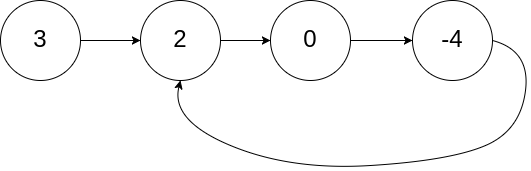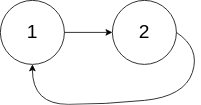# leetcode 209. Minimum Size Subarray Sum

## 题目描述

Given an array of n positive integers and a positive integer s , find
the minimal length of a contiguous subarray of which the sum ≥ s. If
there isn’t one, return 0 instead.

*Example: *

``````Input: s = 7, nums = [2,3,1,2,4,3]
Output: 2
Explanation: the subarray [4,3] has the minimal length under the problem constraint.``````

Follow up:

If you have figured out the O ( n ) solution, try coding another solution
of which the time complexity is O ( n log n ).

Tags: Array, Two Pointers, Binary Search

Difficulty: Medium



# leetcode 125. Valid Palindrome

## 题目描述

Given a string, determine if it is a palindrome, considering only alphanumeric
characters and ignoring cases.

Note: For the purpose of this problem, we define empty string as valid
palindrome.

Example 1:

``````Input: "A man, a plan, a canal: Panama"
Output: true``````

Example 2:

``````Input: "race a car"
Output: false``````

Tags: Two Pointers, String

Difficulty: Easy



# leetcode 15. 3Sum

## 题目描述

Given an array `nums` of n integers, are there elements a , b , c in
`nums` such that a + b + c = 0? Find all unique triplets in the array
which gives the sum of zero.

Note:

The solution set must not contain duplicate triplets.

Example:

``````Given array nums = [-1, 0, 1, 2, -1, -4],

A solution set is:
[
[-1, 0, 1],
[-1, -1, 2]
]``````

Tags: Array, Two Pointers

Difficulty: Medium



# leetcode 844. Backspace String Compare

## 题目描述

Given two strings `S` and `T`, return if they are equal when both are typed
into empty text editors. `#` means a backspace character.

Example 1:

``````Input: S = "ab#c", T = "ad#c"
Output: true
**Explanation** : Both S and T become "ac".``````

Example 2:

``Input: S = "ab``



# leetcode 3. Longest Substring Without Repeating Characters

## 题目描述

Given a string, find the length of the longest substring without repeating
characters.

Example 1:

``````Input: "abcabcbb"
Output: 3
Explanation: The answer is "abc", with the length of 3. ``````

Example 2:

``````Input: "bbbbb"
Output: 1
Explanation: The answer is "b", with the length of 1.``````

Example 3:

``````Input: "pwwkew"
Output: 3
Explanation: The answer is "wke", with the length of 3.
Note that the answer must be a **substring** , "pwke" is a _subsequence_ and not a substring.``````

Tags: Hash Table, Two Pointers, String, Sliding Window

Difficulty: Medium

# leetcode 42. Trapping Rain Water

## 题目描述

Given n non-negative integers representing an elevation map where the width
of each bar is 1, compute how much water it is able to trap after raining.The above elevation map is represented by array [0,1,0,2,1,0,1,3,2,1,2,1]. In
this case, 6 units of rain water (blue section) are being trapped. Thanks
Marcos
for contributing this image!

Example:

``````Input: [0,1,0,2,1,0,1,3,2,1,2,1]
Output: 6``````

Tags: Array, Two Pointers, Stack

Difficulty: Hard

# leetcode 350. Intersection of Two Arrays II

## 题目描述

Given two arrays, write a function to compute their intersection.

Example 1:

``````Input: nums1 = [1,2,2,1], nums2 = [2,2]
Output: [2,2]``````

Example 2:

``````Input: nums1 = [4,9,5], nums2 = [9,4,9,8,4]
Output: [4,9]``````

Note:

• Each element in the result should appear as many times as it shows in both arrays.
• The result can be in any order.

Follow up:

• What if the given array is already sorted? How would you optimize your algorithm?
• What if nums1 ‘s size is small compared to nums2 ‘s size? Which algorithm is better?
• What if elements of nums2 are stored on disk, and the memory is limited such that you cannot load all elements into the memory at once?

Tags: Hash Table, Two Pointers, Binary Search, Sort

Difficulty: Easy

# leetcode 118. Pascal's Triangle

## 题目描述

Given two arrays, write a function to compute their intersection.

Example 1:

``````Input: nums1 = [1,2,2,1], nums2 = [2,2]
Output: [2,2]``````

Example 2:

``````Input: nums1 = [4,9,5], nums2 = [9,4,9,8,4]
Output: [4,9]``````

Note:

• Each element in the result should appear as many times as it shows in both arrays.
• The result can be in any order.

Follow up:

• What if the given array is already sorted? How would you optimize your algorithm?
• What if nums1 ‘s size is small compared to nums2 ‘s size? Which algorithm is better?
• What if elements of nums2 are stored on disk, and the memory is limited such that you cannot load all elements into the memory at once?

Tags: Hash Table, Two Pointers, Binary Search, Sort

Difficulty: Easy

# leetcode 142. Linked List Cycle II

## 题目描述

Given a linked list, return the node where the cycle begins. If there is no
cycle, return `null`.

To represent a cycle in the given linked list, we use an integer `pos` which
represents the position (0-indexed) in the linked list where tail connects to.
If `pos` is `-1`, then there is no cycle in the linked list.

Note: Do not modify the linked list.

Example 1:
Input: head = [3,2,0,-4], pos = 1 Output: tail connects to node index 1 Explanation: There is a cycle in the linked list, where tail connects to the second node.Example 2:
Input: head = [1,2], pos = 0 Output: tail connects to node index 0 Explanation: There is a cycle in the linked list, where tail connects to the first node.Example 3:
Input: head = , pos = -1 Output: no cycle Explanation: There is no cycle in the linked list.Follow-up :
Can you solve it without using extra space?

Tags: Linked List, Two Pointers

Difficulty: Medium

# leetcode 141. Linked List Cycle

## 题目描述

Given a linked list, determine if it has a cycle in it.

To represent a cycle in the given linked list, we use an integer `pos` which
represents the position (0-indexed) in the linked list where tail connects to.
If `pos` is `-1`, then there is no cycle in the linked list.

Example 1:

``````Input: head = [3,2,0,-4], pos = 1
Output: true
Explanation: There is a cycle in the linked list, where tail connects to the second node.``````Example 2:

``````Input: head = [1,2], pos = 0
Output: true
Explanation: There is a cycle in the linked list, where tail connects to the first node.``````Example 3:

``````Input: head = , pos = -1
Output: false
Explanation: There is no cycle in the linked list.``````Follow up:

Can you solve it using O(1) (i.e. constant) memory?

Tags: Linked List, Two Pointers

Difficulty: Easy

:D 一言句子获取中...